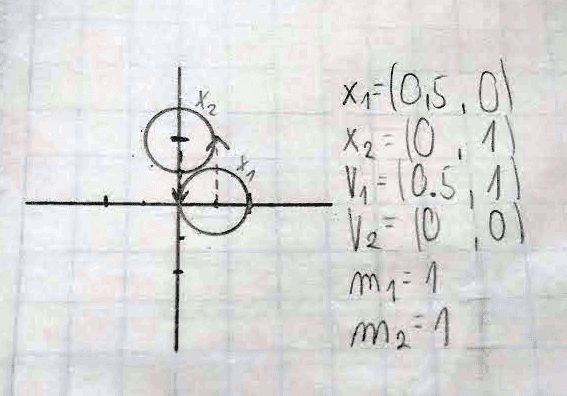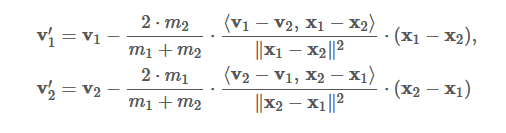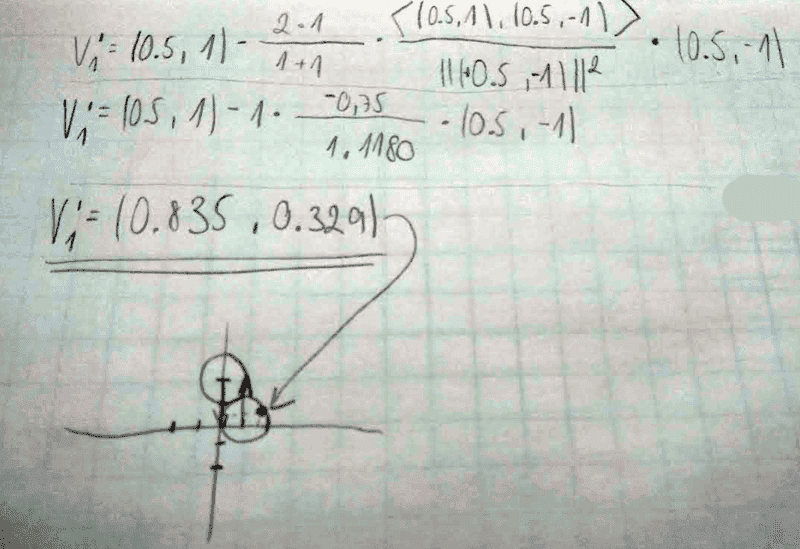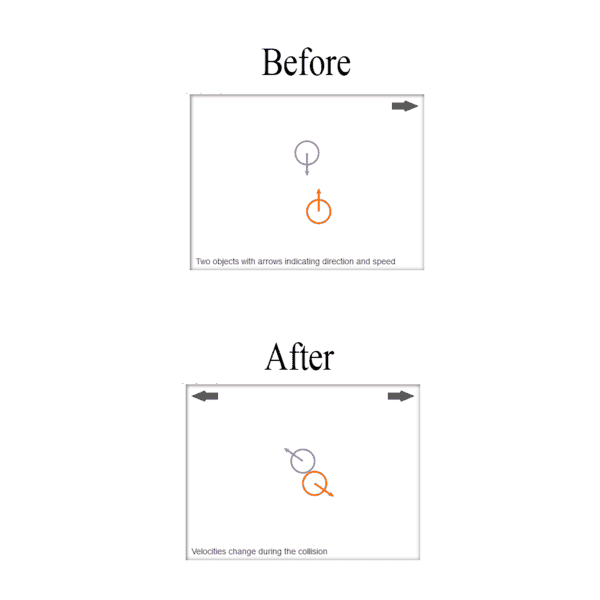# Need help with Elastic collision in 2D

## Homework Statement

Hello im trying to predict vector after collision of 2 ball in biliard. Im using angle-free representation formulas from wikipedia : https://wikimedia.org/api/rest_v1/media/math/render/svg/14d5feb68844edae9e31c9cb4a2197ee922e409c

x1 and x2 are positions of balls, m1 and m2 are masses of balls, v1 and v2 are vectors of direction before collision and the angle brackets indicate the inner product (or dot product) of two vectors.

my values are :
x1 = (0.5 , 0)
x2 = (0 , 1)
m1,m2 = 1
v1 = (0.5, 1)
v2 = (0, 0)## Homework Equations## The Attempt at a Solution[/B]
And here its my solution with vector (0.835 , 0.329), but this result cant be correct can somebody help me what im doing wrong ? My result is total wrong and i dont know why

#### Attachments

BvU
Homework Helper
Hello Matus,Who says it's totally wrong ?
As it happens, the wikipedia page has an animation of 2 5 SF pieces colliding in exactly (##\approx##) this way. You could take screen shots and measure the vectors !

When i simulate my example on some online calculators then vectors must look like this for my parametersBut my final vector after collision its going to wrong direction :(

BvU